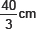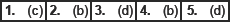# Circles-MCQ

Class X Math
MCQ for CIrcle
1.   In the given figure, PA and PB are tangents from P to a circle with centre O. If ∠AOB = 130°, then find ∠APB.
(a) 40°
(b) 55°
(c) 50°
(d) 60°
2.   In the given figure, PT is a tangent to a circle whose centre is O. If PT = 12 cm and PO = 13 cm then find teh radius of the circle.
(a) 5 cm
(b) 4 cm
(c) 6 cm
(d) 4.5 cm
3.   In the given figure, PT is a tangent to the circle and O is its centre. Find OP.
(a) 16 cm
(b) 15 cm
(c) 18 cm
(d) 17 cm
4.   In the given figure, ABC is a right �� right angled at B such that BC = 6 cm and AB = 8 cm. Find the radius of the circle.
(a) 3 cm
(b) 2 cm
(c) 4 cm
(d) 5 cm
5.   In the figure, Ab is a chord of length 16 cm, of a circle of radius 10 cm. The tangents at A and B intersect at a point P. Find the length of PA.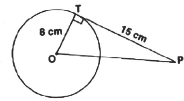(a)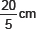(b)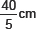(c)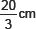(d)